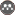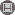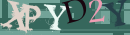Volume 8, Issue 1 (5-2021)                   2021, 8(1): 111-128 | Back to browse issues page

BibTeX | RIS | EndNote | Medlars | ProCite | Reference Manager | RefWorks
Send citation to:Zanganeh Asadi M A, Naemi Tabar M. Relationship between hydrogeomorphic features and suspended sediment load under Kashfarud basins. Journal title 2021; 8 (1) :111-128
URL: http://jsaeh.khu.ac.ir/article-1-3127-en.html
1- Hakim Sabzevari University , Ma.zanganehasadi@hsu.ac.ir
2- Hakim Sabzevari University
Abstract:   (1879 Views)

Relationship between hydrogeomorphic features and suspended sediment load under Kashfarud basins

Introduction
As a stressful stimulus, river sediment is the most significant threat to aquatic ecosystems. To prevent or minimize the damage, three stages of the erosion process should be investigated (Naseri et al., 2019: 83). Determining the amount of sediment transported by rivers is important from different aspects. Sediment carried by water flows is considered a factor effective in shaping the geometric structure and geomorphic characteristics of rivers (Tashekabood et al., 2019: 282).
Data and methodology
To estimate the amount of annual suspended sediments, the flow and sediment statistics of hydrometric stations (8 stations) and meteorological stations (13 stations) were employed (Figure 2). The research statistical period is 25 years (1993-2017). The altitude, area, and perimeter of the basins were obtained from topographic maps with a scale of 1.25000. To investigate the correlation between independent and dependent variables, the normality tests of Shapiro-Wilk and Kolmogorov-Smirnov were performed in SPSS16 software. To extract the geomorphic features of the basins, the digital elevation model was used. Then, ground surface corrections and pretreatments such as removal of hydrological pits were performed and ground drainage pattern was determined.
Stepwise multivariate regression
In the present study, stepwise multivariate regression was used to reduce the number of independent variables and determine the effective factors in the sedimentation of the basin. This method investigates the effect of several independent variables on a dependent variable (Zare Chahuki: 2010). In stepwise multivariate regression, the independent variable that has no more significant effect on the dependent variable is removed from the analysis, hence excluded from the equation. The general form of the stepwise regression equation is:
Equation 1                                                                            Y= a + B1X1 + B2X2 + …… + BnXn + e
Data description and interpretation
The principal component analysis method was used to determine the most effective characteristics of sediments as well as their grouping. In principal component analysis, variables that have a high correlation and are distributed in a multidimensional space are reduced to a set of non-correlated components, each of which is a linear combination of the main variables. The obtained non-correlated components are called principal components (PCs). Prior to component analysis, the KMO coefficient was used to ensure the appropriateness of the data for principal component analysis. This coefficient fluctuates in the range of zero and one and if its value is less than 0.5, the data will not be suitable for principal component analysis and if the values of this coefficient are between 0.5-0.69, The proportionality of the data is moderate and if the value of this coefficient is more than 0.7, the data will be quite suitable for performing principal component analysis.
Regression analysis results
In this study, the sediment weight of the basin was considered as a dependent variable and other parameters as independent variables. The variables of slope, precipitation, basin length, Elongation Ratio (R), circularity coefficient, and unevenness of the basin have a higher correlation with the amount of sediment production in the basin than other variables.
An eigenvalue was used to determine the number of factors. The minimum eigenvalue for the selection of final factors is 1, and factors with an eigenvalue bigger than 1 are considered final factors. The results showed that the three factors of circularity coefficient, compactness coefficient, and basin form coefficient have an eigenvalue bigger than 1.
Conclusion
The results showed that geomorphic parameters have a high correlation with the amount of annual sediment. The results showed that seven factors of slope, precipitation, basin length, elongation ratio, circularity coefficient, unevenness coefficient, and form ratio of the basin were the most important in estimating the amount of suspended sediment based on the principal components analysis method. The average of special sediment varies from 134 tons per year in Dehbar basin to 16 tons per year in Kardeh basin and also the average annual sediment varies from 261.6 tons per year in Golmakan basin to 156.7 tons per year in Shandiz basin. Evaluation of Bartlett's test of sphericity tests and KMO values is 0.9. Therefore, the data is suitable for factor analysis. The percentage of variance explained by each factor indicates that the circularity coefficient with 50.71% of the variance explains all the research variables. In total, three factors of circularity coefficient, compactness coefficient, and form ratio of the basin could explain 82.6% of the variance of all research variables. Therefore, the results are consistent with Lu et al. (1991), Sarangi et al. (2005), Tamene et al. (2006), Zhang et al. (2015), Salim (2014), and Ares et al. (2016).
Khorram Dareh sub-basin with heavy rainfall (504 mm) has the lowest specific sediment, which is due to the geological structure of the region. Based on the calculated indicators, most of the studied sub-basins are elongated. The form ratio of the basin is less indicative of the elongation of the basin. The highest branching ratio of the basins is in the vicinity of faults. Also, high circularity values indicate points prone to sedimentation. River sections up to degree 3 are located in more subdued areas and have a steeper slope. Golmakan, Khorram Darreh, Zashk, and Dehbar sub-basins have a high potential for sedimentation. Regression equations of sediment measurement curves are usually used in sediment load estimates. The most important reason is the ease of application of these equations. According to the research results, it can be concluded that the integrated use of principal component analysis, cluster analysis, and multivariate stepwise regression has a suitable and acceptable efficiency in estimating suspended sediments. Testing the regression model concerning different climatic and hydrological regimes of Iran’s watersheds to achieve an efficient pattern of using these equations can be fruitful in estimating sediment load in different regions.

Keywords: Hydrogeomorphic, Sediment erosion, Kashfarud basin, Stepwise multivariate regression
Type of Study: Research | Subject: Special
Received: 2020/04/30 | Accepted: 2020/09/23 | Published: 2021/06/19Rights and permissionsThis work is licensed under a Creative Commons Attribution-NonCommercial 4.0 International License.
 Rights and permissionsThis work is licensed under a Creative Commons Attribution-NonCommercial 4.0 International License.